# Catch up on the latest AI articles# When And How Should CNN Ensembles Be Used?

3 main points
✔️ Verify whether a single model or an ensemble model is better when the number of parameters is the same
✔️ Experimented and validated the CNN-based image classification task in various settings
✔️ Demonstrate that the ensemble model performs better overall

More or Less: When and How to Build Convolutional Neural Network Ensembles
written by
(Submitted on 29 Sept 2020 (modified: 26 Jan 2021))
Comments: Accepted to ICLR2021.
Subjects: ensemble learning, empirical study, machine learning systems, computer vision## First of all

When you want to improve the performance of a deep learning model, the simplest way is to increase the model size.

But is it better to use a single model with many parameters, or an ensemble of multiple models with relatively few parameters? In the paper presented in this article, we address the question of whether we should use an ensemble or a single model, especially for CNNs, from various perspectives such as training time, inference time, and memory usage.

## Technique

The comparison between ensemble and single models is made from the policies and methods described below.

### The rationale for fair and equitable ranking

As an ensemble/single model comparison, we compare architectures with the same number of parameters. There are two reasons why we did not use other metrics (training time, inference time, memory usage, etc.) instead of the number of parameters here.

• Because the number of parameters in the network is directly proportional to the other indicators.
• The number of parameters does not depend on the hardware used, etc., and can be calculated accurately from the network specifications.

Next, we describe how we design and conduct comparative experiments with single/ensemble network architectures.

### About Single/Ensemble Architecture

To begin, a single CNN architecture is represented by $S^{(w,d)}$. where $w$ is the width of the network, $d$ is the depth, and $|S|$ is the number of parameters.

We also assume that $S^{(w,d)}$ belongs to the class $C$ of neural network architectures. Here, the ensemble network is represented by $E=\{E_1,.... ,E_k\}$. In this case, $E_1,...,E_k ,E_k \in C$ and $|E_1|+... +|E_k|=|S|$. In other words, the class of the architecture is identical to that of a single network, and the total number of parameters is the same.

Also in the experiment, all ensemble network architectures are identical ($E_1=... =R_k$) and the number of parameters is set to be equal ($=|S|/k$). Under these settings, we compare the single network and the ensemble network.

### Depth/width equivalent ensemble

The CNN ensemble network architecture is determined from two different policies: depth equivalent/width equivalent. This is illustrated in the following figure.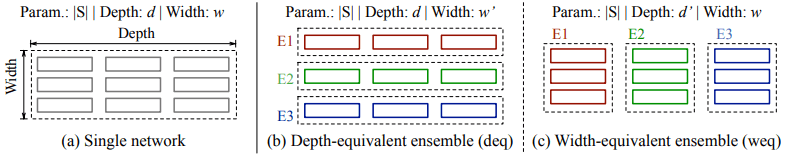Briefly, in the depth-equivalent ensemble setting, the depth of the ensemble network is the same depth $d$ as that of the single network. In the width-equivalent ensemble setting, the width of the ensemble network is the same width $w$ as that of the single network.

In this case, the width $W^{\prime}$ in the depth equivalent setting is set to the maximum value within the range where the total number of parameters does not exceed $|S|$. Similarly, the depth $d^{\prime}$ in the width equivalent setting is set to the maximum value within the range where the total number of parameters does not exceed $|S|$. This is expressed as a mathematical expression as follows.

$w^{\prime}: k \cdot |E^{(w^{\prime},d)}_i| \leq |S^{(w,d)}| \leq k \cdot |E^{(w^{\prime}+1,d)_i}|$

$d^{\prime}: k \cdot |E^{(w,d^{\prime})}_i| \leq |S^{(w,d)}| \leq k \cdot |E^{(w,d^{\prime}+1)_i}|$

Overall, we compare (i) a single network $S^{(w,d)}$ belonging to the same network architecture class $C$, (ii) $k$ width-equivalent ensembles $S^{(w,d^{\prime})}$, and (iii) $k$ depth-equivalent ensembles $S^{(w^{\prime },d)}$ for comparison experiments.

## experiment

### Experiment setup

Data set

The data set used in the experiment is as follows.

• SVHN
• CIFAR-10
• CIFAR-100
• Tiny ImageNet
• Downsampled ImageNet-1k

Architecture

The CNN architecture used in our experiments is as follows.

• VGGNet
• ResNet
• DenseNet
• Wide ResNet

Evaluation index

To compare single network and ensemble network, we evaluate the following five aspects.

• generalization accuracy
• Training time per epoch
• Time to reach the specified accuracy (time to accuracy)
• inference time
• Memory usage

Advanced settings

The hyperparameters and other details of the architecture are as follows. We use PyTorch as the experimental framework and an Nvidia V100 GPU for execution.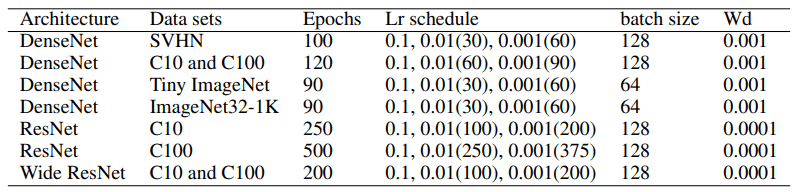## Result

### Accuracy Comparison

The occurrence of EST (Ensemble Switchover Threshold)

The experimental results confirm the phenomenon that both depth/width equivalent ensembles outperform a single network when the resources exceed a certain threshold.

In the paper, we name this EST (Ensemble Switchover Threshold). This is shown in the following figure.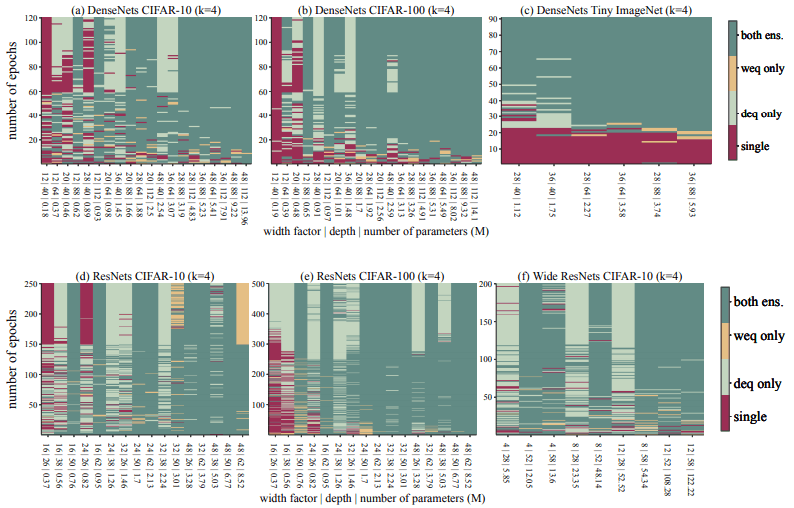In this figure, we visualize which of the single network (single), depth equivalent (deq), and width equivalent (weq) ensembles is dominant. It can be seen that as the number of parameters increases to some extent (to the right), the ensemble method becomes dominant.

Besides, the test error rate in the DenseNet model is as follows.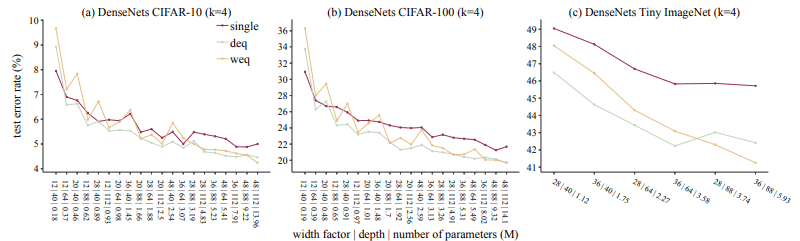Similarly here, when the number of parameters is somewhat larger, the ensemble method is dominant overall. This EST occurs when the resources are low to moderate (about 1M to 1.5M parameters and less than half of the training epoch). Therefore, it can be said that the results show that ensembles may well be more useful even when resources are not present in large quantities.

In general, these results show that ensembles can outperform single networks in a very wide range of use cases.

### For a more effective ensemble

As a result of our experiments, we have observed the following three findings for the effective use of ensemble models.

1. ensembles become more effective the more complex the data set is

The more complex the training dataset is, the closer EST is to the origin (smaller in both the number of epochs and the number of parameters).

2. Increasing the number of ensemble networks is effective under a sufficiently large parameter budget

As shown in the following figure, the larger the number of ensemble networks ($k$), the more the EST moves to the right (requiring more number of parameters).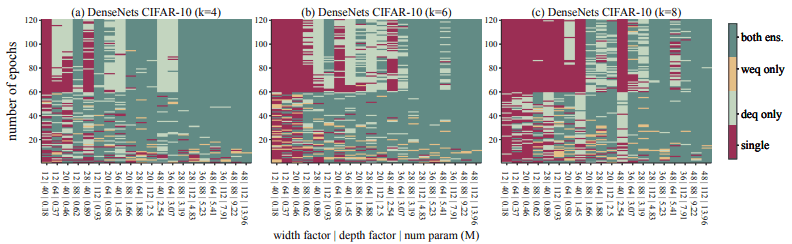Therefore, if we want to increase the number of ensemble networks, we must also ensure a sufficient number of parameters, otherwise, we cannot expect to improve the accuracy.

3. Depth equivalent ensemble shows better accuracy than width equivalent ensemble

For the depth/width equivalent ensemble, we found that the overall accuracy is higher than the depth equivalent ensemble. This can be attributed to the fact that modern CNN architectures are designed to improve accuracy as the depth of the layer increases.

### Comparison of time aspects

Comparison of training time

The training time required to achieve the accuracy of a single network model is shown in the figure below.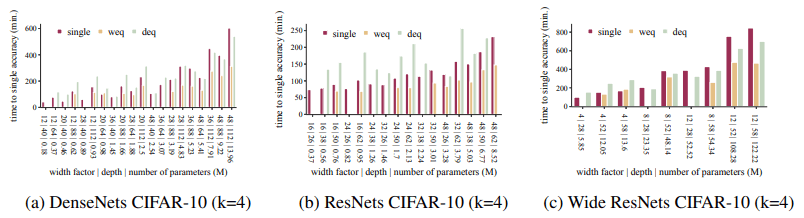(The absence of a bar indicates that the accuracy of the single network model cannot be reached.)

Overall, the ensemble achieves the accuracy of the single network model much faster (1.2 to 5 times in our experiments). Besides, the training time per epoch is as follows.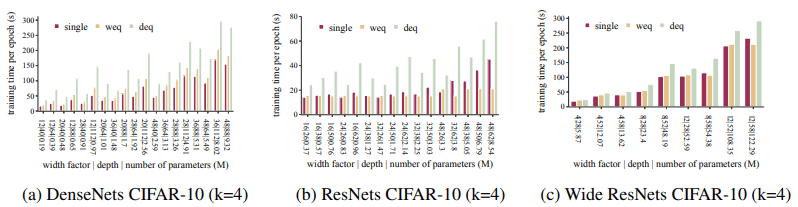Ensembles take longer to train per epoch because they train $k$ networks in both depth/width equivalent cases.

In this case, the depth-equivalent ensemble increases the learning time per epoch by about a factor of two compared to the width-equivalent ensemble. In terms of convergence time, the ensemble converges faster than the single network.

This can be attributed to the fact that all networks in the ensemble are smaller than the single network. In general, the ensemble does not require more training time than the single network, indicating that the ensemble can achieve the accuracy of the single network with less training time.

Comparison of inference time

The following figure shows the inference time per image.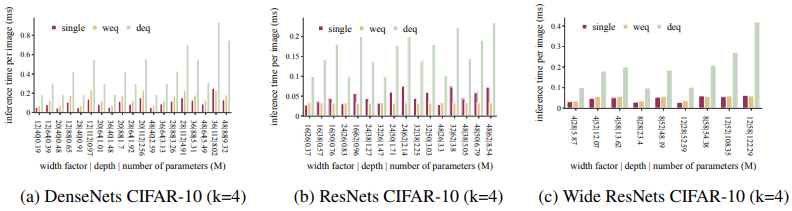For inference time, we see a similar trend as for training time per epoch. That is, the width-equivalent ensemble shows inference speeds comparable to a single network, while the depth-equivalent ensemble is much slower.

### Comparison of memory usage

A comparison of the memory usage for each setting is shown below.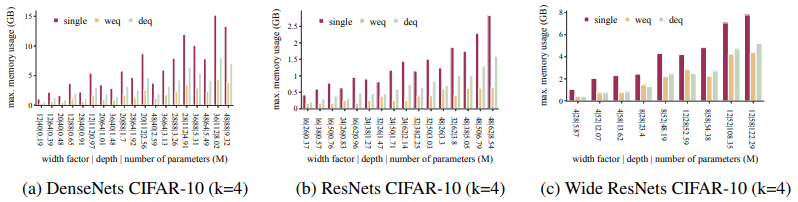This represents the minimum amount of memory required by the GPU in the hyperparameter set described earlier. This result is because the amount of memory required when training an ensemble network is the same as the memory required to train one of the $k$ networks. Advantages of this memory efficiency exist, such as the ability to increase the batch size and the effectiveness of training in memory-constrained environments.

## Summary

In this article, we presented our analysis of the decision on whether or not to use ensembles.

In general, the results show that ensemble models are more accurate than single models when the number of parameters is the same, that they can be trained faster, that they are capable of equivalent inference, and that the amount of memory required can be significantly reduced.

Although there are some limitations in what was verified in the experiment (e.g., the networks in the ensemble must be identical, and the study does not verify anything other than image classification), it is a study that provides very meaningful information.

If you have any suggestions for improvement of the content of the article,
please contact the AI-SCHOLAR editorial team through the contact form.